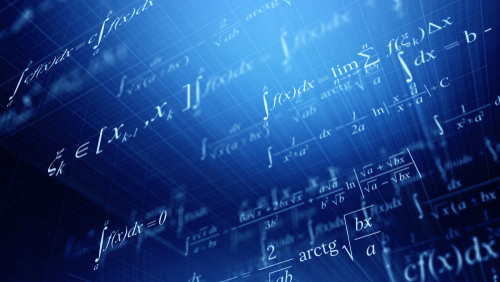# What Types of Math are Most Commonly Used by Data Scientists?Data science is a field that focuses on finding, cleaning, sorting and analyzing data and, therefore, requires knowledge of various types of math. The type of math a data scientist uses will depend largely on the job and employer. A data scientist who is working in the capacity of data engineering will use less math or math that’s slightly different than someone hired as an actual fact-finding data scientist. However, regardless of the job, mathematics is at the center of all scientific fields. There are some types of math that an aspiring data scientist can expect to learn or know.

### Linear Algebra

When we hear the word algebra, what often comes to mind are those annoying formulas we did in high school algebra class. Although data scientists do still use the traditional algebraic formulas, they typically use more complex forms of algebra. Linear algebra, which is the mathematics of data, is one of the most important tools in data science. In fact, it’s the central part of all mathematics. Linear algebra works with mathematical structures, vector spaces, matrices, linear equations, and linear transformations. Unlike traditional algebra, linear algebra deals a lot with lines and provides users with a bunch of mini spreadsheets to help them get their solutions.

### Calculus

Calculus is a very intrinsic type of mathematics and one an individual must know well to be successful in data science. It’s not just that calculus is used a lot in machine learning algorithms but that it’s very important for many key machine learning applications. Calculus can be one of two kinds: integral calculus or differential calculus. Differential calculus will divide something into small pieces, and integral calculus will put the small pieces back together to see what they make and how many there are. Calculus is used by data scientists in almost every model they use.

### Probability

Probability is a branch or type of mathematics that involves calculating the likelihood of some event occurring based on certain variables. More simply explained, probability is the chance or how likely something is going to happen. To determine the likelihood or probability of some event happening, data scientists take the number of different ways an event can happen and divide it by the total number of possible outcomes or P(E) = n(E)/n(T). Probability is important because it helps data scientists make informed decisions on what is likely to happen based on what data has told them in the past.

### Statistics

Statistics is a highly interdisciplinary field that’s vital to data scientists. It involves everything data-related. It’s the practice of collecting data, sifting through the data, getting rid of unnecessary data, sorting and analyzing the remaining, understanding what it means and presenting the findings to others. The purpose of statistics is so data scientists can compare the data and look for specific trends or changes and draw conclusions on what it means. A perfect example is in TV ratings. Statisticians do surveys on what age groups watch certain TV shows and on what nights they get the best viewing. After sorting through all the data, they’re able to determine what day would be the best day to run the program.

### Conclusion

Data scientists are often categorized as mathematicians or statisticians because of the similarities in their jobs and requirements. These professionals are expected to see a job growth of 33% between 2016 and 2026 according to the U.S. Bureau of Labor Statistics. Data scientists with a solid foundation of various types of math should find the most lucrative career choices.

Related Resources: